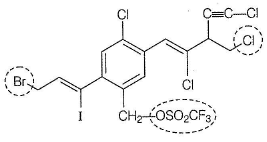Courses

# Test: SN2 Reaction Basics

## 20 Questions MCQ Test Chemistry for JEE | Test: SN2 Reaction Basics

Description
This mock test of Test: SN2 Reaction Basics for NEET helps you for every NEET entrance exam. This contains 20 Multiple Choice Questions for NEET Test: SN2 Reaction Basics (mcq) to study with solutions a complete question bank. The solved questions answers in this Test: SN2 Reaction Basics quiz give you a good mix of easy questions and tough questions. NEET students definitely take this Test: SN2 Reaction Basics exercise for a better result in the exam. You can find other Test: SN2 Reaction Basics extra questions, long questions & short questions for NEET on EduRev as well by searching above.
QUESTION: 1

### Only One Option Correct Type Direction (Q. Nos. 1-8) This section contains 8 multiple choice questions. Each question has four choices (a), (b), (c) and (d), out of which ONLY ONE is correct. Q.  Which of the SN2 reaction is fastest?

Solution:

CH3 Br is more reactive termed and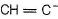is stronger nucleophile.

QUESTION: 2

### Which statement is true about SN2 mechanism?

Solution:

Stronger the nucleophile, faster the SN2 reaction. Polar aprotic solvent solvate cations, makes anionic nucleophile more available for reaction, hence faster reaction. A better leaving group lowers the activation energy increasing rate of SN2 reaction.

QUESTION: 3

### A correct statement about transition state of SN2 reaction is

Solution:

SN2 reaction is a one step (concerted) reaction that involes a single transition state.

QUESTION: 4

What is the correct increasing order of reactivity of the  followings in SN2 reaction ?

I. CH2 = CH — Br
II. CH2 = CH— I
III. CH3CH2CH2 — I
IV. CH3OCH2CH2 — I

Solution:

Allyl bromide (I) is most reactive among the given halides as pi bonds from allylic position stabilises the transition state. Vinyl iodide (II) is least reactive due to partial double bond character. Electron withdrawing inductive effect of CH3O- increases reactivity of (IV) over (III)

QUESTION: 5

What is the correct increasing order of reactivity of the following in the SN2 reaction?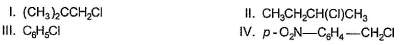Solution:

(IV) is most reactive as it is benzylic as well as electron withdrawing effect of — NO2 further increases the reactivity. (Ill) is least reactive due to resonance effect resulting in partial double bond character between carbon and chlorine. (I) is less reactive in SN2 reaction than II, due to greater steric hindrance in (I).

QUESTION: 6

Consider the two lines shown in the diagram given below.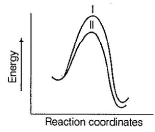Q.

In a SN2 reaction, these two lines compare the effect of the

Solution:

Substrates are same as they are at same potential energies. Nucleophiles are different as magnifested by different activation energies and different products with different potential energies,

QUESTION: 7

Consider the two lines shown in the diagram given below.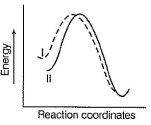Q.

Which of the following apply appropriately to a SN2 reaction?

Solution:

HOis stronger nucleophile than CH3COO- . Hence, lower activation energy of (I) than (II).

QUESTION: 8

The correct statement concerning a SN2 reaction is

Solution:

The transition state in SN2 reaction is pentavalent as indicated here.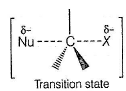*Multiple options can be correct
QUESTION: 9

One or More than One Options Correct Type

Direction (Q. Nos. 9-14) This section contains 6 multiple choice questions. Each question has four choices (a), (b), (c) and (d), out of which ONE or MORE THAN ONE are correct.

Q.

In a SN2 reaction rate of reaction depends on

Solution:

In a SN2 reaction, Rate = k[substrate] [nucleophile]
Hence, rate depends both on concentration of substrate as well as nucleophile. Rate of reaction also depends on nature of leaving group. A better leaving group gives lower activation energy hence, greater rate of reaction. Number of lone pair has no role to play in nucieophilicity hence reactivity.

*Multiple options can be correct
QUESTION: 10

Consider the following SN2 reaction,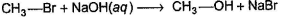Q.

Which of the following could increase the reactivity (rate) of reaction ?

Solution:

Increasing concentration of nucleophile (NaOH) increases the reactivity. Adding some Nal also catalyse reaction because of following equilibrium.Now reaction occur with CH3I which has better leaving group. Heavier isotope forms stronger covalent bond. Hence, replacing CH3Br by 14CH3Br stabilises substrate, decreases rate whereas replacing NaOH by NaO18H stabilises transition state increases rate of reaction.

*Multiple options can be correct
QUESTION: 11

A SN2 reaction involves back side attack of nucleophile at the α-carbon of substrate because

Solution:

Both nucleophile and leaving group are electron rich species, nucleophile attacks from backside (most remote position) to have minimum repulsion with leaving group. Also, due to the presence of leaving group on front side there is less physical space for attack of nucleophile.

*Multiple options can be correct
QUESTION: 12

1-chlorobutane is more reactive than 2-chloro-2-methyl propane in a SN2 reaction because

Solution: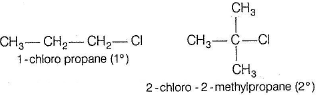As indicated in the given reaction, α-carbon is less hindered in 1- chloropropane. Also + I effect of three methyl groups in 2- chloro-2-methyl propane decreases electropositive character of α-carbon, decreases reactivity further in SN2 reaction.

*Multiple options can be correct
QUESTION: 13

Consider the reaction given below.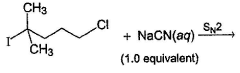Q.

The correct statement(s) applicable to the above reaction is/are

Solution:

Steric hindrance plays the most important role in SN2 reaction. Hence, although Cl is poorer leaving group than I, Cl is substituted predominantly in the above reaction due to less steric hindrance at α-carbon. Addition of Nal replace Cl by I and substitution becomes easier.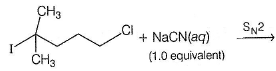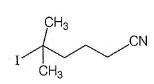*Multiple options can be correct
QUESTION: 14

The correct statement regarding a SN2 reaction is/are

Solution:

Stronger bond formation at α-carbon affects the stability of product (thermodynamics) not the rate (kinetics) of reaction. Change of isotope at α-position or in halogens affect the rate, hence show kinetic isotopic effect. Presence of electron withdrawing group in substrate increases electrophilicity of α-carbon hence, increases its reactivity towards nucleophiles.

QUESTION: 15

Comprehension Type

Direction (Q. Nos. 15-17) This section contains a paragraph, describing theory, experiments, data, etc. Three Questions related to the paragraph have been given. Each question has only one correct answer among the four given options (a), (b), (c) and (d).

Passage

The general mechanism of a SN2 reaction is as follows.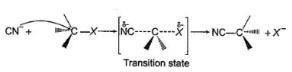Factors that limit the rate of reaction are steric hindrance at α-cabron of substrate and strength of C—X bond. Any factor which stabilises transition state increases the rate of reaction.

Q.

Consider the following SN2 reaction,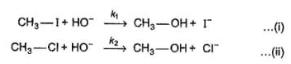Which of the following energy diagram is correctly labelled?

Solution:

Products are same in both reactions, hence same potential energies of products are shown. Also I- is better leaving group, has lower activation energy in SN2 reaction as indicated by curve-l in diagram.

QUESTION: 16

Passage

The general mechanism of a SN2 reaction is as follows.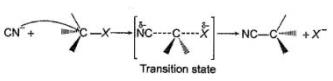Factors that limit the rate of reaction are steric hindrance at α-cabron of substrate and strength of C—X bond. Any factor which stabilises transition state increases the rate of reaction.

Q.

In the previous question, under identical reaction condition, i.e. tem perature, concentration of substrate and nucleophile, the correct relationship between rate constant is

Solution:

Since I- is better leaving group, kI > kII

QUESTION: 17

Passage

The general mechanism of a SN2 reaction is as follows.Factors that limit the rate of reaction are steric hindrance at α-cabron of substrate and strength of C—X bond. Any factor which stabilises transition state increases the rate of reaction.

Q.

Which of the following is not a correct representation of SN2 reaction ?

Solution:

Following neutralisation reaction is preferred over SN2 reaction :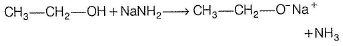*Answer can only contain numeric values
QUESTION: 18

One Integer Value Correct Type

Direction (Q. Nos. 18-20) This section contains 3 questions. When worked out will result in an integer from 0 to 9 (both inclusive).

Consider the following reaction,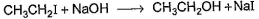Q.

The above reaction was started taking equal concentrations of ethyl iodide and NaOH. After 1.0 h, concentration of iodoethane is dropped to (1/3)rd of initial value. By what factor, the rate of reaction would have been decreased by the same time?

Solution:

Rate = A [C2H5I] [OH-] Rate is linear function of both alkyl halide and nucleophile concentration both will decrease by same factor. Hence, if after 1.0 h concentration of iodoethane decreases to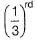concentration of hydroxide will also decrease by the same  factor and rate by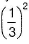, i.e. by 9 times.

*Answer can only contain numeric values
QUESTION: 19

Under identical experimental condition, how many of the following substrate react at faster rate with aqueous NaOH than 2-bromobutane as substrate?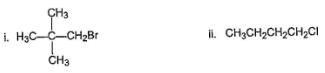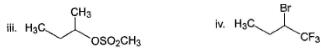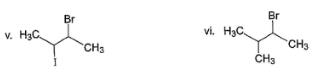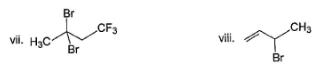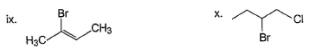Solution:

Compounds (II), (III), (IV), (V), (VIII) and (X) react at faster rate than 2-bromobutane in SN2 reaction.

*Answer can only contain numeric values
QUESTION: 20

Consider the following compound,Q.

If the above compound is treated with excess of NaCN(aq), how many CN- group would be incorporated by SN2 reaction?

Solution:

Only the circled groups are substituted in SN2,